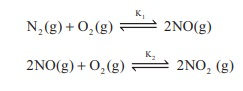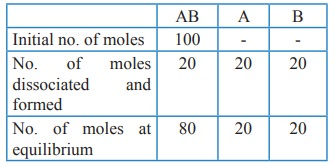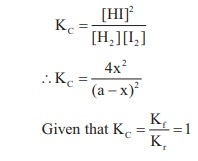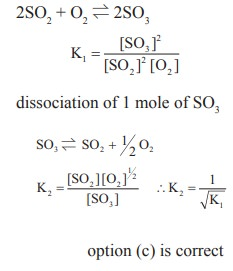Home | | Chemistry 11th std | Choose the best answer: Chemistry: Physical and Chemical Equilibrium

# Choose the best answer: Chemistry: Physical and Chemical Equilibrium

Chemistry : Physical and Chemical Equilibrium - Choose the best answer, Evaluation

Physical and Chemical Equilibrium

Evaluation

1. If Kb and Kf for a reversible reactions are 0.8 ×10–5 and 1.6 × 10–4 respectively, the value of the equilibrium constant is,

a) 20

b) 0.2 × 10–1

c) 0.05

d) none of these

Solution:

Kb = 0.8 × 10–5

Kf = 1.6 × 10–4

Keq = Kf / Kb = 1.6X10-4 / 0.8X10-5 = 20

2.  At a given temperature and pressure, the equilibrium constant values for the equilibriaThe relation between K1 and K2 is

a) K1 = 1/ √K2

b) K2 = (K1)-1/2

c) K12 = 2K2

d) K1/2 = K2

Solution:3.  The equilibrium constant for a reaction at room temperature is K1 and that at 700 K is K2. If K1 > K2, then

a) The forward reaction is exothermic

b) The forward reaction is endothermic

c) The reaction does not attain equilibrium

d) The reverse reaction is exothermic

Solution:

T1 = 25 + 273 = 298 K

T2 = 700 KΔHo is –ve ie., forward reaction is exothermic

4. The formation of ammonia from N2(g) and H2(g) is a reversible reaction

N2(g) + 3H2(g)  2NH3(g) + Heat

What is the effect of increase of temperature on this equilibrium reaction

a) equilibrium is unaltered

b) formation of ammonia is favoured

c) equilibrium is shifted to the left

d) reaction rate does not change

Solution:

Increase in temperature, favours the endothermic reaction,

Given that formation of NH3 is exothermic ie., the reverse reaction is endothermic.

increase in temperature, shift the equilibrium to left option (c)

5. Solubility of carbon dioxide gas in cold water can be increased by

a) increase in pressure

b) decrease in pressure

c) increase in volume

d) none of these

Solution:increase in pressure, favours the forward reaction.

6. Which one of the following is incorrect statement ?

a) for a system at equilibrium, Q is always less than the equilibrium constant

b) equilibrium can be attained from either side of the reaction

c) presence of catalyst affects both the forward reaction and reverse reaction to the same extent

d) Equilibrium constant varied with temperature

Solution:

option (a) : wrong statement

Correct statement is, for a system at equilibrium, Q = Keq

7. K1 and K2 are the equilibrium constants for the reactions respectively.What is the equilibrium constant for the reaction NO2(g)  ½N2(g) + O2(g)

a) 1/√k1√k2

b ) (K1=K2)1/2

c)  1/2K1K2

d) (1 / K1K2)1/2

Solution:8. In the equilibrium,

2A(g)  2B(g) + C2(g)

the equilibrium concentrations of A, B and C2 at 400 K are 1 × 10–4 M, 2.0 × 10–3 M, 1.5 × 10–4 M respectively. The value of KC for the equilibrium at 400 K is

a) 0.06

b) 0.09

c) 0.62

d) 3 × 10–2

Solution:

[A] = 1 × 10–4 M ;

[B] = 2 × 10–3 M

[C] = 1.5 × 10–4 M

2A (g)  2B(g) + C2(g)9. An equilibrium constant of 3.2 × 10–6 for a reaction means, the equilibrium is

a) largely towards forward direction

b) largely towards reverse direction

c) never established

d) none of these

Solution:

Kc = [Products] / [Reactants]

3.2 x 10-6 = [Products] / [Reactants]

KC < 10-3 ; indicates

that [Reactant] >> [Product]

option (b) is correct,largely towards reverse direction.

10. KC/ KP for the reaction, N2(g) + 3H2(g)  2NH3(g) is

a) 1 / RT

b) √(RT)

c) RT

d) (RT)2

Solution:

for the reaction,

N2(g) + 3H2(g)  2NH3(g) ;

Δng = 2 – 4 = – 2

KP = KC (RT)–2

Kc/Kp = (RT)2

11. For the reaction AB (g)  A(g) + B(g), at equilibrium, AB is 20% dissociated at a total pressure of P, The equilibrium constant KP is related to the total pressure by the expression

a) P = 24 KP

b) P = 8 KP

c) 24 P = KP

d) none of these

Solution:Total no. of moles at equilibrium = 80 + 20 + 20 = 12012. In which of the following equilibrium, KP and KC are not equal?

a) 2 NO(g)  N2(g) + O2(g)

b) SO2 (g) + NO2  SO3(g) + NO(g)

c) H2(g) + I2(g)  2HI(g)

d) PCl5 (g)  PCl3(g) + Cl2(g)

Solution:

For reaction given in option (a), (b) & (c) Δng = 0

For option (d) Δng = 2 – 1 = 1

KP = KC (RT)

13. If x is the fraction of PCl5 dissociated at equilibrium in the reaction

PCl5  PCl3 + Cl2

then starting with 0.5 mole of PCl5, the total number of moles of reactants and products at equilibrium is

a) 0.5 – x

b) x + 0.5

c) 2x + 0.5

d) x + 1

Solution:Total no. of moles at equilibrium = 0.5–x + x + x

= 0.5 + x

14. The values of K P1 and KP2 for the reactions

Y + Z

2B are in the ratio 9 : 1 if degree of dissociation and initial concentration of X and A be equal then total pressure at equilibrium P1, and P2 are in the ratio

a) 36 : 1

b) 1 : 1

c) 3 : 1

d) 1 : 9

### Solution:15. In the reaction,

Fe (OH)3 (s)  Fe3+(aq) + 3OH(aq),

if the concentration of OH ions is decreased by ¼ times, then the equilibrium concentration of Fe3+ will

a) not changed

b) also decreased by ¼ times

c) increase by 4 times

d) increase by 64 times

### Solution:To maintain KC as constant, concentration of Fe3+ will increase by 64 times.

16. Consider the reaction where KP = 0.5 at a particular temperature

PCl5(g)  PCl3 (g) + Cl2 (g)

if the three gases are mixed in a container so that the partial pressure of each gas is initially 1 atm, then which one of the following is true

a) more PCl3 will be produced

b) more Cl2 will be produced

c) more PCl5 will be produced

d) none of these

### Solution:

KP = 0.5Q > KP  Reverse reaction is favoured ; i.e. more PCl5 will be produced. option (c)

17. Equimolar concentrations of H2 and I2 are heated to equilibrium in a 1 litre flask. What percentage of initial concentration of H2 has reacted at equilibrium if rate constant for both forward and reverse reactions are equal

a) 33%

b) 66%

c) (33)2 %

d) 16.5 %

### Solution:

V = 1L

H2 + I2  2HI

[H2]initial = [I2]initial = a

[H2]eq = [I2]eq = (a – x)

and [HI]eq = 2x4x2 = (a – x)2

4x2 = a2 + x2 – 2ax

3x2 + 2ax – a2 = 0

x = –a & x = a/3

degree of dessociation = a/3× 100

= 33.33 %

18. In a chemical equilibrium, the rate constant for the forward reaction is 2.5 × 102 and the equilibrium constant is 50. The rate constant for the reverse reaction is,

a) 11.5

b) 5

c) 2 × 102

d) 2 × 10–3

### Solution:

Kf = 2.5 × 102

KC = 50

Kr = ?

Kc = Kf/Kr

50 = 2.5 x 102/ Kr

Kr = 5

19. Which of the following is not a general characteristic of equilibrium involving physical process

a) Equilibrium is possible only in a closed system at a given temperature

b) The opposing processes occur at the same rate and there is a dynamic but stable condition

c) All the physical processes stop at equilibrium

d) All measurable properties of the system remains constant

### Solution:

correct statement : Physical processes occurs at the same rate at equilibrium

option (c) is incorrect statement

20. For the formation of Two moles of SO3(g) from SO2 and O2, the equilibrium constant is K1. The equilibrium constant for the dissociation of one mole of SO3 into SO2 and O2 is

a) 1 /K1

b ) K12

c) (1/K1)1/2

d) K1/2

### Solution:21. Match the equilibria with the corresponding conditions,

i) Liquid  Vapour

ii) Solid  Liquid

iii) Solid  Vapour

iv) Solute (s)  Solute (Solution)

1. melting point

2. Saturated solution

3. Boiling point

4. Sublimation point

5. Unsaturated solution

a) i:1, ii:2, iii:3, iv:4

b) i:3, ii:1, iii:4, iv:2

c) i:2, ii:1, iii:3, iv:4

d) i:3, ii:2, iii:4, iv:5

22. Consider the following reversible reaction at equilibrium, A + B  C, If the concentration of the reactants A and B are doubled, then the equilibrium constant will

a) be doubled

b) become one fourth

c) be halved

d) remain the same

### Solution:

A + B  C

KC= [C] / [A] [B]

if [A] and [B] are doubled, [C] increases 4 times to maintain KC as constant.

equilibrium constant will remain the same – option (d)

23. [Co(H2O)6]2+ (aq) (pink) + 4Cl (aq)  [CoCl4]2– (aq) (blue)+ 6 H2O (l)

In the above reaction at equilibrium, the reaction mixture is blue in colour at room temperature. On cooling this mixture, it becomes pink in colour. On the basis of this information, which one of the following is true ?

a) ΔH > 0 for the forward reaction

b) ΔH = 0 for the reverse reaction

c) ΔH < 0 for the forward reaction

d) Sign of the ΔH cannot be predicted based on this information.

### Solution:

on cooling, reverse reaction predominates and the solution is pink in colour.

decrease in temperature, favours the reverse reaction ie reverse reaction is exothermic and for the forward reaction is endothermic (ΔH > 0)

24. The equilibrium constants of the following reactions are :

N2 + 3H2  2NH3 ; K1

N2 + O2  2NO ; K2

H2 + ½O2  H2O ; K3

The equilibrium constant (K) for the reaction ;

2NH3 + 5/2 O2 ↔k↔ 2NO+3H2O+3H2O, will be

a) K23K/ k1

b) K2K33 / k2

c) K2K33 / k1

d) K2K/ k1

### Solution:25. A 20 litre container at 400 K contains CO2 (g) at pressure 0.4 atm and an excess of SrO (neglect the volume of solid SrO). The volume of the container is now decreased by moving the movable piston fitted in the container. The maximum volume of the container, when pressure of CO2 attains its maximum value will be :

Given that : SrCO3 (S)  SrO (S) + CO2(g)

KP = 1.6 atm

a) 2 litre

b) 5 litre

c) 10 litre

d) 4 litre

### Solution:

Given that KP = 1.6 atm

V1 = 20 L

V2 = ?

T1 = 400 K

T2 = 400 K

KP = PCO2

PCO2= 1.6 atm

P1 = 0.4 atm. P2 = 1.6 atmTags : Multiple choice questions with answers, Solution and Explanation , 11th Chemistry : UNIT 8 : Physical and Chemical Equilibrium
Study Material, Lecturing Notes, Assignment, Reference, Wiki description explanation, brief detail
11th Chemistry : UNIT 8 : Physical and Chemical Equilibrium : Choose the best answer: Chemistry: Physical and Chemical Equilibrium | Multiple choice questions with answers, Solution and Explanation